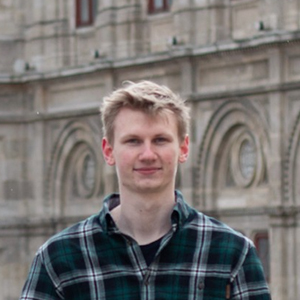blog.hirnschall.net#### Subscribe for New Articles

You can clearly see the cut where the gif starts from the beginning which is something we don't want. As we are using 2d perlin nosie to generate this animation you should take a look at this guide to perlin-noise if you are not yet familiar with it.

You can see side by side which section of perlin noise we are using and the corresponding animation frame in fig. 1.2.

To alleviate this problem we can move the section of noise we work with in a circle instead of a straight line. You can see the result below:

Although there is no longer an obvious cut when the video restarts, the problem is that we are now scrolling sideways and the animation is no longer the same.

To overcome this problem we will take the idea of moving in a circle to three dimensions or in general, if we are using $$n$$-dimensional perlin noise in the original animation we'll now use $$n+1$$ dimensions.

So what we'll do is mapping our flat canvas to the outside of a cylinder in 3d space. By doing so we know there won't be any cuts as we are going in a circle but the animation will stay the same! We can do this by mapping the each point $$(x,y)$$ to $$(x',y',z')$$:

$$x'=\sin\left(\frac{x\cdot 2\pi}{U}\right)\cdot R$$ $$y'=y$$ $$z'=\begin{cases} \sqrt{R^2-x'^2} & \text{if \frac{6\pi}{4} < (\frac{x\cdot 2\pi}{U} mod 2\pi) <\frac{2\pi}{4}} \\ -\sqrt{R^2-x'^2} & \text{otherwise} \end{cases}$$

Where $$U$$ is the circumference and $$R$$ is the radius of the cylinder. By changing the size of the cylinder we can adjust the length of the final animation.

As (in p5js at least) $$noise(x)=noise(-x)$$ we will offset the center of our cylinder from $$0$$. This can be done by adding $$R$$ to each coordinate. So, we'll use $$noise(R+x',R+y',R+z')$$ instead of $$noise(x,y)$$.

You can see the section of noise we are using for the final animation in fig. 1.4 below. However it is hard to visualize 3d noise correctly..

And the final animation without any jumps or cuts:

Feel free to share your results as well as questions below. I am eager to see how you use this technique!Article by: Sebastian Hirnschall
Updated: 03.03.2021